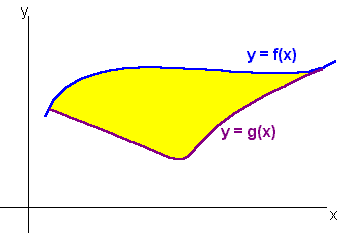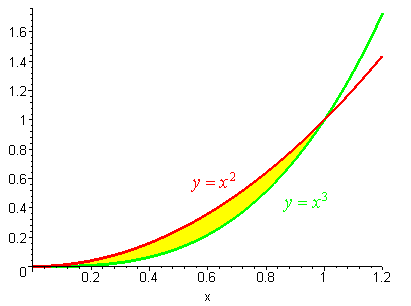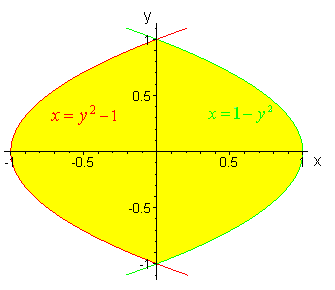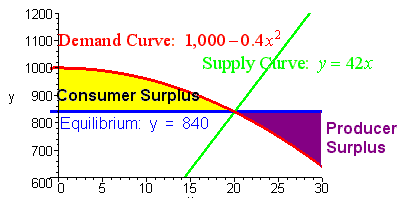$$\newcommand{\id}{\mathrm{id}}$$ $$\newcommand{\Span}{\mathrm{span}}$$ $$\newcommand{\kernel}{\mathrm{null}\,}$$ $$\newcommand{\range}{\mathrm{range}\,}$$ $$\newcommand{\RealPart}{\mathrm{Re}}$$ $$\newcommand{\ImaginaryPart}{\mathrm{Im}}$$ $$\newcommand{\Argument}{\mathrm{Arg}}$$ $$\newcommand{\norm}{\| #1 \|}$$ $$\newcommand{\inner}{\langle #1, #2 \rangle}$$ $$\newcommand{\Span}{\mathrm{span}}$$

# 1.1: Area Between Two Curves

$$\newcommand{\vecs}{\overset { \rightharpoonup} {\mathbf{#1}} }$$ $$\newcommand{\vecd}{\overset{-\!-\!\rightharpoonup}{\vphantom{a}\smash {#1}}}$$$$\newcommand{\id}{\mathrm{id}}$$ $$\newcommand{\Span}{\mathrm{span}}$$ $$\newcommand{\kernel}{\mathrm{null}\,}$$ $$\newcommand{\range}{\mathrm{range}\,}$$ $$\newcommand{\RealPart}{\mathrm{Re}}$$ $$\newcommand{\ImaginaryPart}{\mathrm{Im}}$$ $$\newcommand{\Argument}{\mathrm{Arg}}$$ $$\newcommand{\norm}{\| #1 \|}$$ $$\newcommand{\inner}{\langle #1, #2 \rangle}$$ $$\newcommand{\Span}{\mathrm{span}}$$ $$\newcommand{\id}{\mathrm{id}}$$ $$\newcommand{\Span}{\mathrm{span}}$$ $$\newcommand{\kernel}{\mathrm{null}\,}$$ $$\newcommand{\range}{\mathrm{range}\,}$$ $$\newcommand{\RealPart}{\mathrm{Re}}$$ $$\newcommand{\ImaginaryPart}{\mathrm{Im}}$$ $$\newcommand{\Argument}{\mathrm{Arg}}$$ $$\newcommand{\norm}{\| #1 \|}$$ $$\newcommand{\inner}{\langle #1, #2 \rangle}$$ $$\newcommand{\Span}{\mathrm{span}}$$

Recall that the area under a curve and above the x-axis can be computed by the definite integral. If we have two curves

$$y = f(x)$$ and $$y=g(x)$$

such that

$f(x) > g(x) \nonumber$then the area between them bounded by the horizontal lines $$x = a$$ and $$x = b$$ is

$\text{Area}=\int_{c}^{b} \left [ f(x) - g(x) \right ] \;dx. \nonumber$

To remember this formula we write

$\text{Area}=\int_{a}^{b}\text{(Top-Bottom)}\;dx \nonumber$

Example 1

Find the area between the curves $$y=x^2$$ and $$y=x^3$$.Solution

First we note that the curves intersect at the points $$(0,0)$$ and $$(1,1)$$. Then we see that

$x^3 < x^2 \nonumber$

in this interval. Hence the area is given by

\begin{align*} \int_{0}^{1} \left( x^2 - x^3 \right) dx &= {\left[ \frac{1}{3}x^3 - \frac{1}{4}x^4 \right]}_0^1 \\ &= \dfrac{1}{3} - \dfrac{1}{4} \\ &= \dfrac{1}{12}. \end{align*}

## Area Bounded by Two Functions of $$y$$

Example 2

Find the area between the curves $$x = 1 - y^2$$ and $$x = y^2-1$$.

Solution

Here the curves bound the region from the left and the right.We use the formula

$\text{Area}=\int_{c}^{b}\text{(Right-Left)}\;dy. \nonumber$

For our example:

\begin{align*} \int_{-1}^{1}\big[ (1-y^2)-(y^2-1) \big] dy &= \int_{-1}^{1}(2-y^2) dy \\ &= \left(2y-\dfrac{2}{3}y^3\right]_{-1}^1 \\ &=\big(2-\dfrac{2}{3}\big)-\big(-2-\dfrac{2}{3} \big) \\ &= \dfrac{8}{3}. \end{align*}

Example 3

Find the area between the curves $$y =0$$ and $$y = 3 \left( x^3-x \right)$$.

Solution

When we graph the region, we see that the curves cross each other so that the top and bottom switch. Hence we split the integral into two integrals:\begin{align*} \int_{-1}^{0}\big[ 3(x^3-x)-0\big] dx +\int_{0}^{1}\big[0-3(x^3-x) \big] dx &= \left(\dfrac{3}{4}x^4-\dfrac{3x^2}{2}\right]_{-1}^0 - \left(\dfrac{3}{4}x^4-\dfrac{3x^2}{2}\right]_0^1 \\ &=\big(-\dfrac{3}{4}+\dfrac{3}{2} \big) - \big(\dfrac{3}{4}-\dfrac{3}{2} \big) \\ &=\dfrac{3}{2} \end{align*}.

## Application

Let $$y = f(x)$$ be the demand function for a product and $$y = g(x)$$ be the supply function. Then we define the equilibrium point to be the intersection of the two curves. The consumer surplus is defined by the area above the equilibrium value and below the demand curve, while the producer surplus is defined by the area below the equilibrium value and above the supply curve.

Example 4

Find the producer surplus for the demand curve

$f(x) = 1000 - 0.4x^2 \nonumber$

and the supply curve of

$g(x) = 42x. \nonumber$

SolutionWe first find the equilibrium point:

We set

$1000 - 0.4x^2 = 42x \nonumber$

or

$0.4x^2 + 42x - 1000 = 0. \nonumber$

We get

$x=20 \nonumber$

hence

$y=42(20)=840. \nonumber$

We integrate

\begin{align*} \int_{0}^{20} \left ( 840 - 42x \right ) dx &= {\left[ 840x-21x^2 \right] }_0^{20} \\[4pt] &= 8400. \end{align*}

Exercises

1. Find the area between the curves $$y = x^2$$ and $$y =\sqrt{x}$$.
2. Find the area between the curves $$y = x^2 - 4$$ and $$y = -2x$$.
3. Find the area between the curves $$y = 2/x$$ and $$y = -x + 3$$.
4. Find the area between the curves $$y = x3^x$$ and $$y = 2x +1$$.